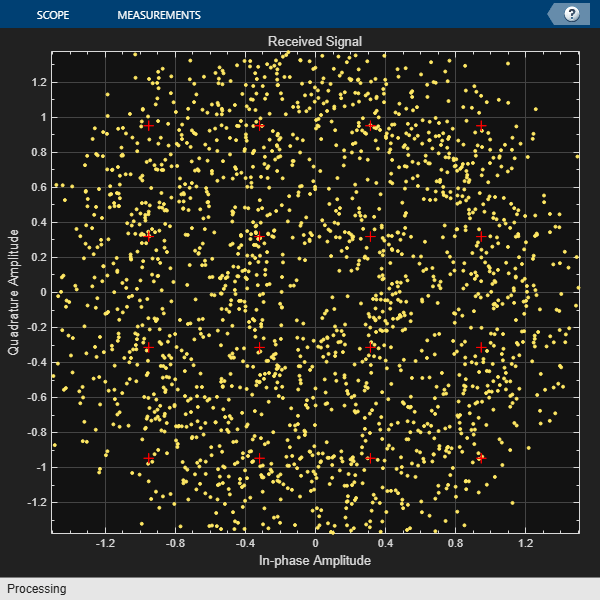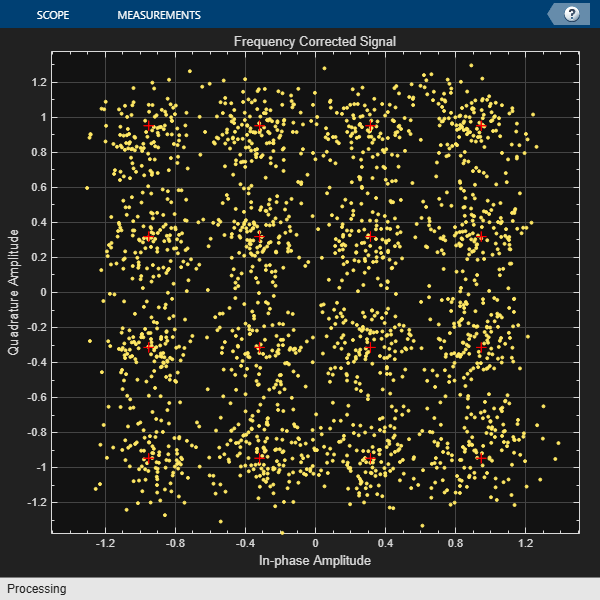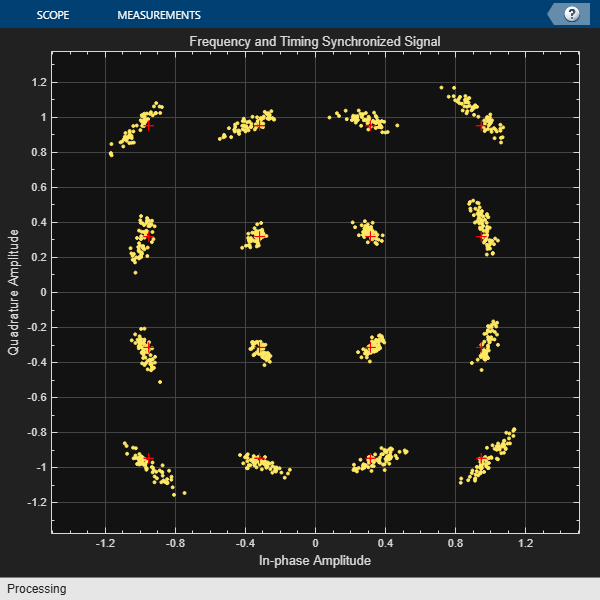# Correct Symbol Timing and Doppler Offsets

Correct symbol timing and frequency offset errors by using the `comm.SymbolSynchronizer` and `comm.CarrierSynchronizer` System objects.

### Configuration

Initialize simulation parameters.

```M = 16; % Modulation order nSym = 2000; % Number of symbols in a packet sps = 2; % Samples per symbol spsFilt = 8; % Samples per symbol for filters and channel spsSync = 2; % Samples per symbol for synchronizers lenFilt = 10; % RRC filter length```

Create a matched pair of root raised cosine (RRC) filter System objects for transmitter and receiver.

```txfilter = comm.RaisedCosineTransmitFilter( ... FilterSpanInSymbols=lenFilt, ... OutputSamplesPerSymbol=spsFilt, ... Gain=sqrt(spsFilt)); rxfilter = comm.RaisedCosineReceiveFilter( ... FilterSpanInSymbols=lenFilt, ... InputSamplesPerSymbol=spsFilt, ... DecimationFactor=spsFilt/2, ... Gain=sqrt(1/spsFilt));```

Create a phase-frequency offset System object to introduce a 100 Hz Doppler shift.

```doppler = comm.PhaseFrequencyOffset( ... FrequencyOffset=100, ... PhaseOffset=45, ... SampleRate=1e6);```

Create a variable delay System object to introduce timing offsets.

`varDelay = dsp.VariableFractionalDelay;`

Create carrier and symbol synchronizer System objects to correct for Doppler shift and timing offset, respectively.

```carrierSync = comm.CarrierSynchronizer( ... SamplesPerSymbol=spsSync); symbolSync = comm.SymbolSynchronizer( ... TimingErrorDetector='Early-Late (non-data-aided)', ... SamplesPerSymbol=spsSync);```

Create constellation diagram System objects to view the results.

```refConst = qammod(0:M-1,M,UnitAveragePower=true); cdReceive = comm.ConstellationDiagram( ... ReferenceConstellation=refConst, ... SamplesPerSymbol=spsFilt,Title='Received Signal'); cdDoppler = comm.ConstellationDiagram( ... ReferenceConstellation=refConst, ... SamplesPerSymbol=spsSync, ... Title='Frequency Corrected Signal'); cdTiming = comm.ConstellationDiagram( ... ReferenceConstellation=refConst, ... SamplesPerSymbol=spsSync, ... Title='Frequency and Timing Synchronized Signal');```

### Main Processing Loop

The main processing loop:

• Generates random symbols and applies QAM modulation.

• Filters the modulated signal.

• Applies frequency and timing offsets.

• Passes the transmitted signal through an AWGN channel.

• Corrects the Doppler shift.

• Corrects the timing offset.

```for k = 1:15 data = randi([0 M-1],nSym,1); modSig = qammod(data,M,UnitAveragePower=true); txSig = txfilter(modSig); txDoppler = doppler(txSig); txDelay = varDelay(txDoppler,k/15); rxSig = awgn(txDelay,25); rxFiltSig = rxfilter(rxSig); rxCorr = carrierSync(rxFiltSig); rxData = symbolSync(rxCorr); end```

### Visualization

Plot the constellation diagrams of the received signal, frequency corrected signal, and frequency and timing synchronized signal. Specific constellation points cannot be identified in the received signal and can be only partially identified in the frequency corrected signal. However, the timing and frequency synchronized signal aligns with the expected QAM constellation points.

`cdReceive(rxSig)``cdDoppler(rxCorr)``cdTiming(rxData)`